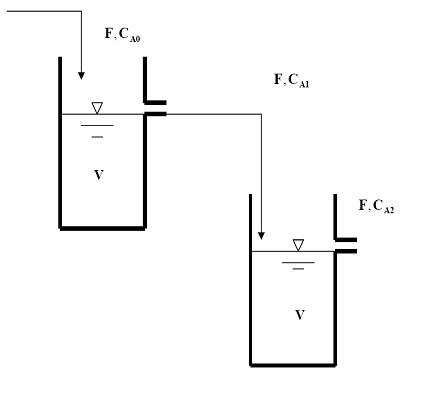Documentation

This example shows how to design a compensator in an IMC structure for series chemical reactors, using Control System Designer. Model-based control systems are often used to track setpoints and reject load disturbances in process control applications.

### Plant Model

The plant for this example is a chemical reactor system, comprised of two well-mixed tanks.The reactors are isothermal and the reaction in each reactor is first order on component A:

``

Material balance is applied to the system to generate a dynamic model of the system. The tank levels are assumed to stay constant because of the overflow nozzle and hence there is no level control involved.

For details about this plant, see Example 3.3 in Chapter 3 of "Process Control: Design Processes and Control Systems for Dynamic Performance" by Thomas E. Marlin.

The following differential equations describe the component balances:

``
``

``
``

the material balances are:

``
``

where,, andare steady-state values.

Substitute, the following design specifications and reactor parameters:

``
``
``
``

The resulting steady-state concentrations in the two reactors are:

``
``

where

``

For this example, design a controller to maintain the outlet concentration of reactant from the second reactor,, in the presence of any disturbance in feed concentration,. The manipulated variable is the molar flowrate of the reactant, `F`, entering the first reactor.

### Linear Plant Models

In this control design problem, the plant model is

``

and the disturbance model is

``

This chemical process can be represented using the following block diagram:where

``
``
``
``

Based on the block diagram, obtain the plant and disturbance models as follows:

``
``

Create the plant model at the command line:

```s = tf('s'); G1 = (13.3259*s+3.2239)/(8.2677*s+1)^2; G2 = G1; Gd = 0.4480/(8.2677*s+1)^2; ```

G1 is the real plant used in controller evaluation. G2 is an approximation of the real plant and it is used as the predictive model in the IMC structure. `G2 = G1` means that there is no model mismatch. `Gd` is the disturbance model.

### Define IMC Structure in Control System Designer

Open Control System Designer.

`controlSystemDesigner`Select the IMC control architecture. In Control System Designer, click Edit Architecture. In the Edit Architecture dialog box, select Configuration 5.Load the system data. For G1, G2, and Gd, specify a model Value.### Tune Compensator

Plot the open-loop step response of `G1`.

```step(G1) ```Right-click the plot and select Characteristics > Rise Time submenu. Click the blue rise time marker.The rise time is about 25 seconds and we want to tune the IMC compensator to achieve a faster closed-loop response time.

To tune the IMC compensator, in Control System Designer, click Tuning Methods, and select Internal Model Control (IMC) Tuning.Select a Dominant closed-loop time constant of `2` and a Desired controller order of `2`.To view the closed-loop step response, in Control System Designer, double-click the IOTransfer_r2y:step plot tab.### Control Performance with Model Mismatch

When designing the controller, we assumed G1 was equal to G2. In practice, they are often different, and the controller needs to be robust enough to track setpoints and reject disturbances.

Create model mismatches between G1 and G2 and examine the control performance at the MATLAB command line in the presence of both setpoint change and load disturbance.

Export the IMC Compensator to the MATLAB workspace. Click Export. In the Export Model dialog box, select compensator model C.Click Export.

Convert the IMC structure to a classic feedback control structure with the controller in the feedforward path and unit feedback.

```C = zpk([-0.121 -0.121],[-0.242, -0.466],2.39); C_new = feedback(C,G2,+1) ```
```C_new = 2.39 (s+0.121)^4 --------------------------------------------- (s-0.0001594) (s+0.121) (s+0.1213) (s+0.2419) Continuous-time zero/pole/gain model. ```

Define the following plant models:

• No Model Mismatch:

```G1p = (13.3259*s+3.2239)/(8.2677*s+1)^2; ```
• `G1` time constant changed by 5%:

```G1t = (13.3259*s+3.2239)/(8.7*s+1)^2; ```
• `G1` gain is increased by 3 times:

```G1g = 3*(13.3259*s+3.2239)/(8.2677*s+1)^2; ```

Evaluate the setpoint tracking performance.

```step(feedback(G1p*C_new,1),feedback(G1t*C_new,1),feedback(G1g*C_new,1)) legend('No Model Mismatch','Mismatch in Time Constant','Mismatch in Gain') ```Evaluate the disturbance rejection performance.

```step(Gd*feedback(1,G1p*C_new),Gd*feedback(1,G1t*C_new),Gd*feedback(1,G1g*C_new)) legend('No Model Mismatch','Mismatch in Time Constant','Mismatch in Gain') ```The controller is fairly robust to uncertainties in the plant parameters.